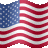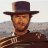# Calculate 5 minute Heikin Ashi on 1 minute chart

J

#### JoshF

##### New member
Hello,

I am trying to develop an indicator based on the 1 minute Heikin Ashi with the 5 minute Heikin Ashi bar as confirmation of trend. I am trying to determine how I can calculate the 5 minute HA values from within the 1 minute chart - anyone able to help? I have the following code which uses the two aggregations, but this is not allowed when creating alerts or scans. So I am looking for some help on how I would manually calculate the 5 minute using the 1 minute data and not reference two different time periods:

Code:
``````#### Start Agg1

input agg1 = AggregationPeriod.min;

def o1 = open(period = agg1);
def h1 = high(period = agg1);
def l1 = low(period = agg1);
def c1 = close(period = agg1);

def haclose = (o1+h1+l1+c1)/4;
def haOpen = if haOpen == 0 then haClose else (haOpen + haClose) / 2;
def haHigh = Max(high, Max(haClose, haOpen));
def haLow = Min(low, Min(haClose, haOpen));

def trend1 = if haClose > haOpen then 1 else if haClose < haOpen then -1 else 0;

#### End AggTime1

#### Start AggTime2
input agg2 = AggregationPeriod.Five_min;

def o2 = open(period = agg2);
def h2 = high(period = agg2);
def l2 = low(period = agg2);
def c2 = close(period = agg2);

def haclose2 = (o2+h2+l2+c2)/4;
def haOpen2 = if haOpen2 == 0 then haClose2 else (haOpen2 + haClose2) / 2;
def haHigh2 = Max(high, Max(haClose2, haOpen2));
def haLow2 = Min(low, Min(haClose2, haOpen2));

def trend2 = if haClose2 > haOpen2 then 1 else if haClose2 < haOpen2 then -1 else 0;``````How to calculate tomorrows close price Questions 21Can a study be made to calculate volume within a certain time bracket? Questions 1